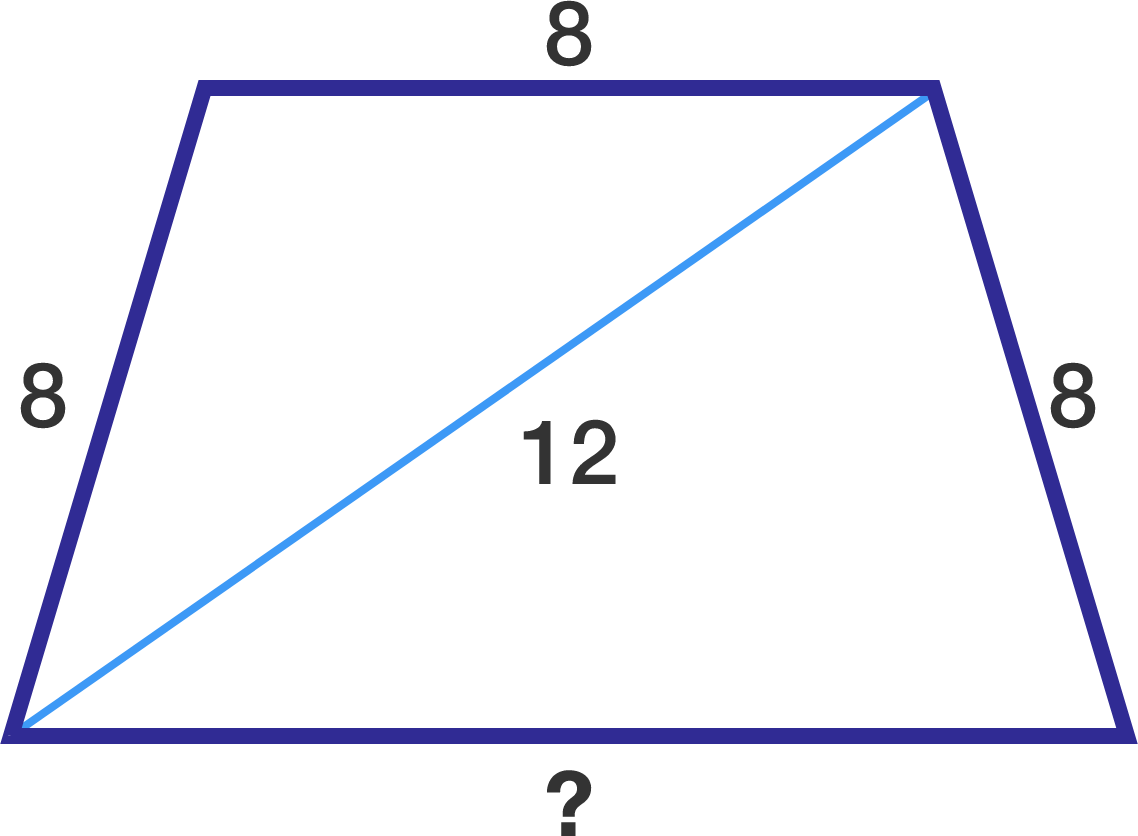# A geometry problem by Julian Yu

Geometry Level 3

I have a trapezoid which has a diagonal of length 12 and three sides with the same length 8.

If $A$ denotes the area of this trapezoid, what is $A^2?$×